## 如何查找在Excel中使用某個命名範圍的位置？

####使用“查找和替換”功能查找在某個命名範圍內使用的位置

1。 按 按Ctrl + F 同時按下鍵以打開“查找和替換”對話框。

2.在打開的“查找和替換”對話框中，請執行以下顯示的屏幕截圖：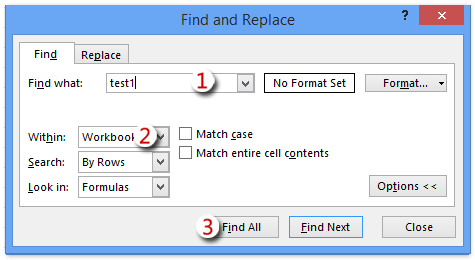（1）將特定命名範圍的名稱鍵入 查找內容 框;

（2）選擇 工作簿 來自 下拉列表;

（3）點擊 找到所有 按鈕。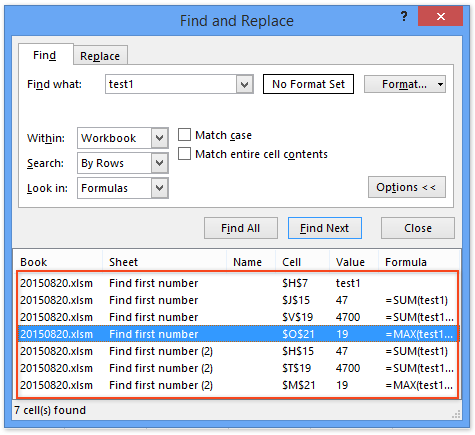####查找VBA使用特定命名範圍的位置

1。 按 其他 + F11 同時按下鍵以打開“ Microsoft Visual Basic for Applications”窗口。

2。 點擊 插入 > 模塊，然後將以下代碼複製並粘貼到打開的“模塊”窗口中。

VBA：查找使用特定命名範圍的位置

``````Sub Find_namedrange_place()
Dim xRg As Range
Dim xCell As Range
Dim xSht As Worksheet
Dim xFoundAt As String
Dim xShName As String
Dim xSearchName As String
On Error Resume Next
xShName = Application.InputBox("Please type a sheet name you will find cells in:", "Kutools for Excel", Application.ActiveSheet.Name)
Set xSht = Application.Worksheets(xShName)
Set xRg = xSht.Cells.SpecialCells(xlCellTypeFormulas)
On Error GoTo 0
If Not xRg Is Nothing Then
xSearchName = Application.InputBox("Please type the name of named range:", "Kutools for Excel")
Set xCell = xRg.Find(What:=xSearchName, LookIn:=xlFormulas, _
LookAt:=xlPart, SearchOrder:=xlByRows, SearchDirection:=xlNext, _
MatchCase:=False, SearchFormat:=False)
If Not xCell Is Nothing Then
If IsPresent(xCell.Formula, xSearchName) Then
End If
Do
Set xCell = xRg.FindNext(xCell)
If Not xCell Is Nothing Then
If IsPresent(xCell.Formula, xSearchName) Then
If xFoundAt = "" Then
Else
xFoundAt = xFoundAt & ", " & xCell.Address
End If
End If
Else
Exit Do
End If
Loop
End If
If xFoundAt = "" Then
Else
MsgBox "The Named Range has been found these locations: " & xFoundAt, , "Kutools for Excel"
End If
On Error Resume Next
xSht.Range(xFoundAt).Select
End If
End Sub
Private Function IsPresent(sFormula As String, sName As String) As Boolean
Dim xPos1 As Long
Dim xPos2 As Long
Dim xLen As Long
Dim I As Long
xLen = Len(sFormula)
xPos2 = 1
Do
xPos1 = InStr(xPos2, sFormula, sName) - 1
If xPos1 < 1 Then Exit Do
IsPresent = IsVaildChar(sFormula, xPos1)
xPos2 = xPos1 + Len(sName) + 1
If IsPresent Then
If xPos2 <= xLen Then
IsPresent = IsVaildChar(sFormula, xPos2)
End If
End If
Loop
End Function
Private Function IsVaildChar(sFormula As String, Pos As Long) As Boolean
Dim I As Long
IsVaildChar = True
For I = 65 To 90
If UCase(Mid(sFormula, Pos, 1)) = Chr(I) Then
IsVaildChar = False
Exit For
End If
Next I
If IsVaildChar = True Then
If UCase(Mid(sFormula, Pos, 1)) = Chr(34) Then
IsVaildChar = False
End If
End If
If IsVaildChar = True Then
If UCase(Mid(sFormula, Pos, 1)) = Chr(95) Then
IsVaildChar = False
End If
End If
End Function``````
3。 點擊 按鈕或按下 F5 運行此VBA的密鑰。

4.現在首開 Kutools for Excel 對話框，請在其中輸入工作表名稱，然後單擊 OK 按鈕; 然後在第二個打開的對話框中，輸入某些命名範圍的名稱，然後單擊 OK 按鈕。 查看屏幕截圖：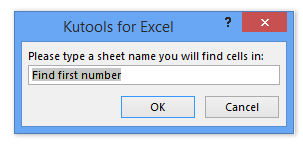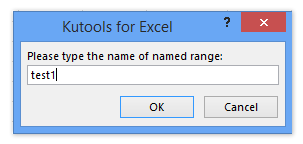5.現在是第三個 Kutools for Excel 出現對話框並使用特定命名範圍列出單元格，如下圖所示。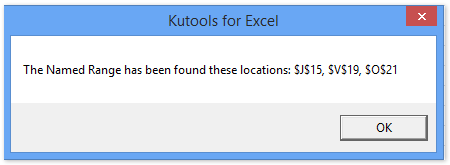####查找某個命名範圍的使用位置 Kutools for Excel

Kutools for Excel - 包含 300 多個 Excel 基本工具。 享受功能齊全的 30- 天免費試用，無需信用卡！ 現在下載！

1。 點擊 庫工具 > 更多 > 替換範圍名稱 打開“替換範圍名稱”對話框。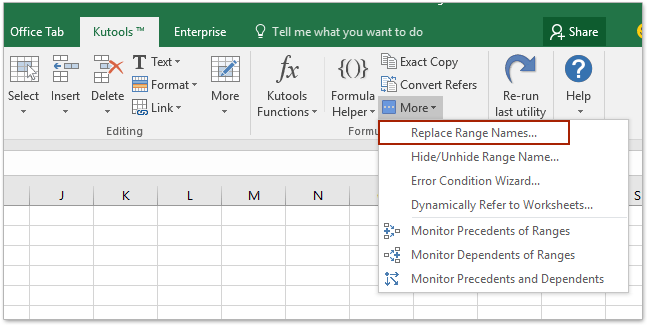2.在打開的“替換範圍名稱”對話框中，轉到 姓名 選項卡，然後單擊 基本名稱 下拉列表，然後從中選擇特定的命名範圍，如下圖所示：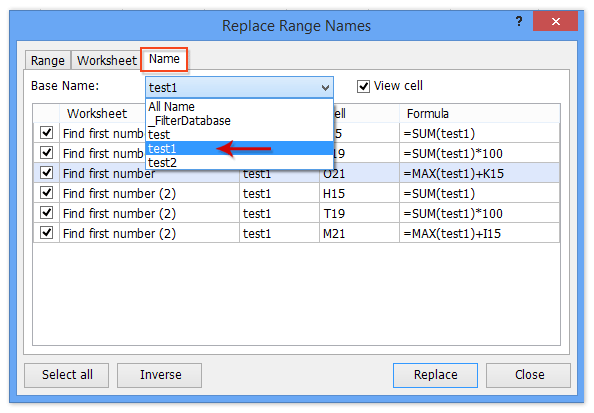3.關閉“替換範圍名稱”對話框。

Kutools for Excel - 使用 300 多種基本工具增強 Excel 功能。 享受功能齊全的 30- 天免費試用，無需信用卡！ 立即行動吧!

Kutools for Excel：超過 300 個方便的工具觸手可及！ 開始你的 30今天免費試用一天，沒有任何功能限制。 現在就下載！

### 最佳辦公生產力工具

 熱門特色: 尋找、突出顯示或識別重複項   |  刪除空白行   |  合併列或儲存格而不遺失數據   |   沒有公式的回合 ... 超級查詢: 多條件VLookup  |   多值VLookup  |   跨多個工作表的 VLookup   |   模糊查詢 .... 高級下拉列表: 快速建立下拉列表   |  依賴下拉列表   |  多選下拉列表 .... 欄目經理: 新增特定數量的列  |  移動列  |  切換隱藏列的可見性狀態  |  比較範圍和列 ... 特色功能: 網格焦點   |  設計圖   |   大方程式酒吧   |  工作簿和工作表管理器   |  資源庫 （自動文字）   |  日期選擇器   |  合併工作表   |  加密/解密單元格   |  按清單發送電子郵件   |  超級濾鏡   |   特殊過濾器 （過濾粗體/斜體/刪除線...）... 前 15 個工具集:  12 文本 工具 (添加文本, 刪除字符，...）   |   50+ 圖表 類型 (甘特圖，...）   |   40+ 實用 公式 (根據生日計算年齡，...）   |   19 插入 工具 (插入二維碼, 從路徑插入圖片，...）   |   12 轉化 工具 (數字到單詞, 貨幣兌換，...）   |   7 合併與拆分 工具 (高級合併行, 分裂細胞，...）   |   ... 和更多#### Office Tab 為 Office 帶來選項卡式界面，讓您的工作更輕鬆

• 在Word，Excel，PowerPoint中啟用選項卡式編輯和閱讀，發布者，Access，Visio和Project。
• 在同一窗口的新選項卡中而不是在新窗口中打開並創建多個文檔。
• 將您的工作效率提高 50%，每天為您減少數百次鼠標點擊！